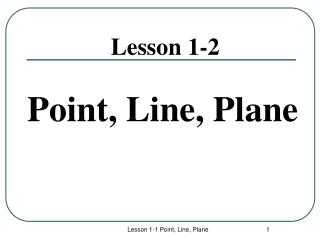DownloadDownload PresentationLesson 1-2

# Lesson 1-2

Download Presentation## Lesson 1-2

- - - - - - - - - - - - - - - - - - - - - - - - - - - E N D - - - - - - - - - - - - - - - - - - - - - - - - - - -
##### Presentation Transcript

1. Lesson 1-2 Point, Line, Plane Lesson 1-1 Point, Line, Plane

2. Objectives What we will learn • We will learn to identify and draw models of points, lines, and planes, and determine their characteristics. Lesson 1-1 Point, Line, Plane

3. Points • Points do not have actual size. • How to Sketch: Using dots • How to label: Use capital letters Never name two points with the same letter (in the same sketch). A B A C Lesson 1-1 Point, Line, Plane

4. Lines • Lines extend indefinitely and have no thickness or width. • How to sketch : using arrows at both ends. • How to name: 2 ways (1) small script letter – line n (2) any two points on the line – AB, BC, AC • Never name a line using three points - n A B C Lesson 1-1 Point, Line, Plane

5. Line Segment • Line segments are straightand they have twoendpoints. • NO arrows on both ends! • Line segments are part of a line, and come in various lengths. • Below is a picture of a line segment. When we draw one, we usually use dots to emphasize the endpoints. Lesson 1-1 Point, Line, Plane

6. Line Segment • How to name: using their endpoints. A or B Lesson 1-1 Point, Line, Plane

7. Ray • A ray is straight and it has one endpoint. • A ray extends indefinitely (forever) in one direction. Endpoint Lesson 1-1 Point, Line, Plane

8. Ray • How to name: using its endpoint and any other point on the ray. Note: We always write the endpoint first, then the other point. CD C D Lesson 1-1 Point, Line, Plane

9. Collinear Points • Collinear points are points that lie on the same line. (The line does not have to be visible.) • A point lies on the line if the coordinates of the point satisfy the equation of the line. Ex: To find if A (1, 0) is collinear with the points on the line y = -3x + 3. Substitute x = 1 and y = 0 in the equation. 0 = -3 (1) + 3 0 = -3 + 3 0 = 0 The point A satisfies the equation, therefore the point is collinear with the points on the line. A B C Collinear C A B Non collinear Lesson 1-1 Point, Line, Plane

10. Planes • A plane is a flat surface that extends indefinitely in all directions. • How to sketch: Use a parallelogram (four sided figure) • How to name: 2 ways (1) Capital script letter – Plane M (2) Any 3 non collinear points in the plane - Plane: ABC/ ACB / BAC / BCA / CAB / CBA A M B C Horizontal Plane Vertical Plane Other Lesson 1-1 Point, Line, Plane

11. Different planes in a figure: A B Plane ABCD Plane EFGH Plane BCGF Plane ADHE Plane ABFE Plane CDHG Etc. D C E F H G Lesson 1-1 Point, Line, Plane

12. Name the planes Front: 1) 2) Back: 1) 2) Top: 1) 2) Bottom: 1) 2) Left: 1) 2) Right: 1) 2) Lesson 1-1 Point, Line, Plane

13. Other planes in the same figure: Any three non collinear points determine a plane! Plane AFGD Plane ACGE Plane ACH Plane AGF Plane BDG Etc. Lesson 1-1 Point, Line, Plane

14. Coplanar Objects Coplanar objects (points, lines, etc.) are objects that lie on the same plane. The plane does not have to be visible. Are the following points coplanar? A, B, C ? Yes A, B, C, F ? No H, G, F, E ? Yes E, H, C, B ? Yes A, G, F ? Yes C, B, F, H ? No Lesson 1-1 Point, Line, Plane

15. Lesson 1-3 Postulates Lesson 1-1 Point, Line, Plane

16. Objectives: What we’ll learn… To identify and use basic postulates about points, lines, and planes. Lesson 1-1 Point, Line, Plane

17. Definition: Postulate (Axiom): Statements in geometry that are accepted as true. Lesson 1-1 Point, Line, Plane

18. Postulate 1-1: Two points determine a unique line. m Q P There is only one line that contains point P and Q. Continued……. Lesson 1-1 Point, Line, Plane

19. Postulate 1-2: The intersection of two lines is a point. m Line m and line n intersect at point P. P n Continued……. Lesson 1-1 Point, Line, Plane

20. Postulate 1-3: Three noncollinear points determine a unique plane. Ç There is only one plane that contains points A, B , and C. B A Continued……. Lesson 1-1 Point, Line, Plane

21. Postulate 1-4 Intersection of Two Planes is a LINE. B P A R Plane P and Plane R intersect at the line Lesson 1-1 Point, Line, Plane

22. 3 Possibilities of Intersection of a Line and a Plane (1) Line passes through plane – intersection is a point. (2) Line lies on the plane - intersection is a line. (3) Line is parallel to the plane - no common points. Lesson 1-1 Point, Line, Plane